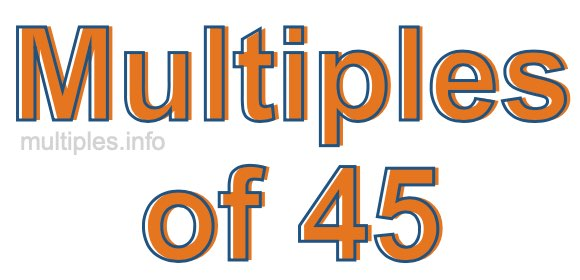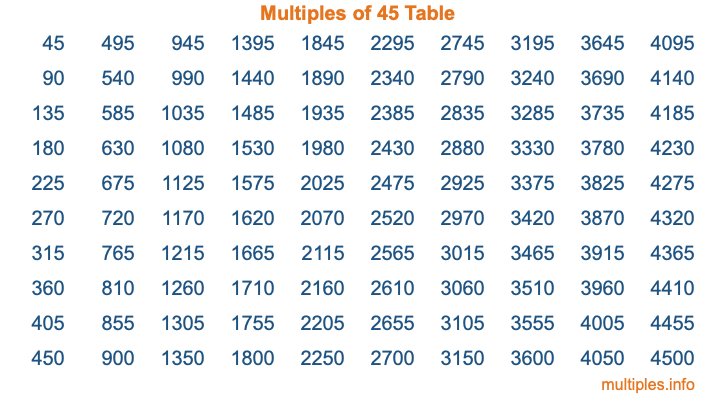Multiples of 45Welcome to the Multiples of 45 page. Here we will first teach you everything you will ever need to know about the multiples of 45, and then give you a study guide summary of everything we taught you to make sure you remember it all. Use this page to look up facts and learn information about the multiples of 45. This page will make you a multiples of forty-five expert!

Definition of Multiples of 45
Multiples of 45 are all the numbers that when divided by 45 equal an integer. Each of the multiples of 45 are called a multiple. A multiple of 45 is created by multiplying 45 by an integer.

Therefore, to create a list of multiples of 45, you start with 1 multiplied by 45, then 2 multiplied by 45, then 3 multiplied by 45, and so on for as long as you want. Thus, the list of the first five multiples of 45 is 45, 90, 135, 180, and 225. To see a larger list of multiples of 45, see the printable image of Multiples of 45 further down on this page. We also have a category where you can choose any nth multiple of 45.

Multiples of 45 Checker
The Multiples of 45 Checker below checks to see if any number of your choice is a multiple of 45. In other words, it checks to see if there is any number (integer) that when multiplied by 45 will equal your number. To do that, we divide your number by 45. If the the quotient is an integer, then your number is a multiple of 45.

Is  a multiple of 45?

Least Common Multiple of 45 and ...
A Least Common Multiple (LCM) is the lowest multiple that two or more numbers have in common. This is also called the smallest common multiple or lowest common multiple and is useful to know when you are adding our subtracting fractions. Enter one or more numbers below (45 is already entered) to find the LCM.

Check out our LCM Calculator if you need more details about the Least Common Multiple or if you need the LCM for different numbers for adding and subtraction fractions.

nth Multiple of 45
As we stated above, 45 is the first multiple of 45, 90 is the second multiple of 45, 135 is the third multiple of 45, and so on. Enter a number below to find the nth multiple of 45.

th multiple of 45

Multiples of 45 vs Factors of 45
45 is a multiple of 45 and a factor of 45, but that is where the similarities end. All postive multiples of 45 are 45 or greater than 45. All positive factors of 45 are 45 or less than 45.

Below is the beginning list of multiples of 45 and the factors of 45 so you can compare:

Multiples of 45: 45, 90, 135, 180, 225, etc.

Factors of 45: 1, 3, 5, 9, 15, 45

As you can see, the multiples of 45 are all the numbers that you can divide by 45 to get a whole number. The factors of 45, on the other hand, are all the whole numbers that you can multiply by another whole number to get 45.

It's also interesting to note that if a number (x) is a factor of 45, then 45 will also be a multiple of that number (x).

Multiples of 45 vs Divisors of 45
The divisors of 45 are all the integers that 45 can be divided by evenly. Below is a list of the divisors of 45.

Divisors of 45: 1, 3, 5, 9, 15, 45

The interesting thing to note here is that if you take any multiple of 45 and divide it by a divisor of 45, you will see that the quotient is an integer.

Multiples of 45 Table
Below is an image of the first 100 multiples of 45 in a table. The table is in chronological order, column by column. The first column has the first ten multiples of 45, the second column has the next ten multiples of 45, and so on.The Multiples of 45 Table is also referred to as the 45 Times Table or Times Table of 45. You are welcome to print out our table for your studies.

Negative Multiples of 45
Although not often discussed or needed in math, it is worth mentioning that you can make a list of negative multiples of 45 by multiplying 45 by -1, then by -2, then by -3, and so on, to get the following list of negative multiples of 45:

-45, -90, -135, -180, -225, etc.

Multiples of 45 Summary
Below is a summary of important Multiples of 45 facts that we have discussed on this page. To retain the knowledge on this page, we recommend that you read through the summary and explain to yourself or a study partner why they hold true.

There are an infinite number of multiples of 45.

A multiple of 45 divided by 45 will equal a whole number.

45 divided by a factor of 45 equals a divisor of 45.

The nth multiple of 45 is n times 45.

The largest factor of 45 is equal to the first positive multiple of 45.

45 is a multiple of every factor of 45.

45 is a multiple of 45.

A multiple of 45 divided by a divisor of 45 equals an integer.

45 divided by a divisor of 45 equals a factor of 45.

Any integer times 45 will equal a multiple of 45.

Multiples of a Number
Here you can get the multiples of another number, all with the same attention to detail as we did for multiples of 45 on this page.

Multiples of
Multiples of 46
Did you find our page about multiples of forty-five educational? Do you want more knowledge? Check out the multiples of the next number on our list!